International
Tables for
Crystallography
Volume B
Reciprocal space
Edited by U. Shmueli

International Tables for Crystallography (2006). Vol. B, ch. 5.1, pp. 541-542   | 1 | 2 |

## Section 5.1.6.1. Absorption coefficient

A. Authiera*

aLaboratoire de Minéralogie-Cristallographie, Université P. et M. Curie, 4 Place Jussieu, F-75252 Paris CEDEX 05, France
Correspondence e-mail: authier@lmcp.jussieu.fr

#### 5.1.6.1. Absorption coefficient

| top | pdf |

In transmission geometry, the imaginary part of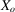is small and, using a first-order approximation for the expansion of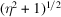, (5.1.5.1)and (5.1.5.2), the effective absorption coefficient in the absorption case is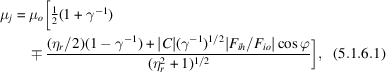where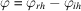is the phase difference between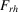and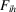[equation (5.1.2.10)], the upper sign (−) for the ∓ term corresponds to branch 1 and the lower sign (+) corresponds to branch 2 of the dispersion surface. In the symmetric Laue case (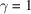, reflecting planes normal to the crystal surface), equation (5.1.6.1)reduces to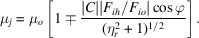Fig. 5.1.6.1shows the variations of the effective absorption coefficient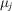with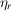for wavefields belonging to branches 1 and 2 in the case of the 400 reflection of GaAs with Cu Kα radiation. It can be seen that for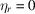the absorption coefficient for branch 1 becomes significantly smaller than the normal absorption coefficient,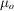. The minimum absorption coefficient,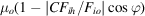, depends on the nature of the reflection through the structure factor and on the temperature through the Debye–Waller factor included in[equation (5.1.2.10b)] (Ohtsuki, 1964, 1965). For instance, in diamond-type structures, it is smaller for reflections with even indices than for reflections with odd indices. The influence of temperature is very important when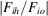is close to one; for example, for germanium 220 and Mo Kα radiation, the minimum absorption coefficient at 5 K is reduced to about 1% of its normal value,(Ludewig, 1969).Figure 5.1.6.1 | top | pdf |Variation of the effective absorption with the deviation parameter in the transmission case for the 400 reflection of GaAs using Cu Kα radiation. Solid curve: branch 1; broken curve: branch 2.

### References

Ludewig, J. (1969). Debye–Waller factor and anomalous absorption (Ge; 293–5 K). Acta Cryst. A25, 116–118.Google Scholar
Ohtsuki, Y. H. (1964). Temperature dependence of X-ray absorption by crystals. I. Photo-electric absorption. J. Phys. Soc. Jpn, 19, 2285–2292.Google Scholar
Ohtsuki, Y. H. (1965). Temperature dependence of X-ray absorption by crystals. II. Direct phonon absorption. J. Phys. Soc. Jpn, 20, 374–380.Google Scholar